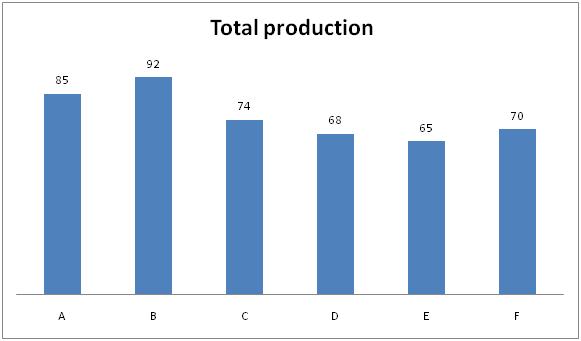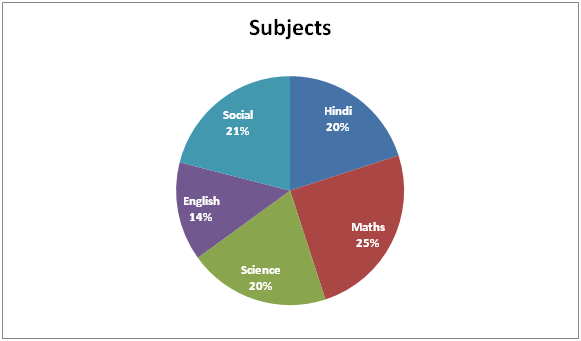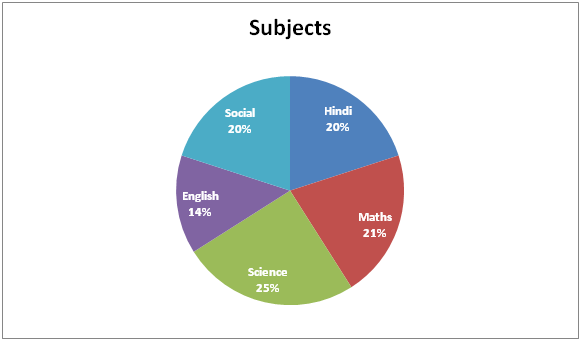# Quantitative Aptitude Questions (Data Interpretation) for Indian Bank PO prelims 2018 Day-171

Dear Readers, Indian Bank is conducting Online Examination for the recruitment of Probationary Officer. To enrich your preparation here we have providing new series of Data Interpretation – Quantitative Aptitude Questions. Candidates those who are appearing in Indian Bank PO prelims Exams can practice these Quantitative Aptitude average questions daily and make your preparation effective.

[WpProQuiz 3290]

Click “Start Quiz” to attend these Questions and view Solutions

Directions (1 – 5): Study the following information carefully and answer the given questions.

Following bar graph shows the total production (In thousands) of six different companies in June 2018.1) If the total production of company B in July 2018 is increased by 56 % while comparing with the previous month, then find the ratio between the total production of company B in July 2018 to that of company D and E in June 2018?

a) 1752 : 1855

b) 2157 : 1652

c) 3588 : 3325

d) 526 : 429

e) None of these

2) If the sales of Company A and C in June 2018 is 78 % and 86 % respectively, then find the average sales of company A and C in June 2018?

a) 64970

b) 52780

c) 48960

d) 40650

e) None of these

3) Find the difference between the total production of company A, C and E together to that of total production of company B, D and F together?

a) 7000

b) 8500

c) 5500

d) 6000

e) None of these

4) Company E and F have produced type 1 and type 2 items in June 2018. If the ratio of type 1 to that of type 2 items in company E is 6 : 7 and the type 1 item of company F is 25 % of type 2 item of company F in June 2018, then find the sum of type 1 items in company E and F together?

a) 56000

b) 44000

c) 46000

d) 38000

e) None of these

5) Total production of company A is approximately what percentage of total production of company E?

a) 130 %

b) 85 %

c) 110 %

d) 70 %

e) 95 %

Directions (6 – 10): Study the following pie chart carefully to answer the questions below:

Following Pie chart1 shows the percentage of students passed in different subjects.

Total student = 8000Pie chart2 shows the percentage of girls passed in different subjects out of the total students.

Total number of girls = 50006) Find the pass percentage of boys in English?

a) 36 %

b) 37.5 %

c) 38 %

d) 37 %

e) 36.5 %

7) How many boys are passed in Science and Social together?

a) 1045

b) 1050

c) 1060

d) 1030

e) 1020

8) Find the average number of girls passed in Hindi, Maths and Science together?

a) 2050

b) 2150

c) 1100

d) 2000

e) 1550

9) Number of boys passed in Hindi is what percentage less than the number of Girls passed in Science?

a) 30 %

b) 35 %

c) 40 %

d) 52 %

e) 64 %

10) What is the respective ratio of number of boys passed in English to the number of boys passed in Social?

a) 21 : 34

b) 34 : 21

c) 31 : 24

d) 24 : 31

e) None of these

The total production of company B in July 2018

= > 92*(156/100) = 143.52 thousands = 143520

The total production of company D and E in June 2018

= > 68 + 65 = 133 thousands = 133000

Required ratio = 143520 : 133000 = 3588 : 3325

The sales of company A in June 2018 = 85000*(78/100) = 66300

The sales of company C in June 2018 = 74000*(86/100) = 63640

The average sales of company A and C in June 2018

= > (66300 + 63640)/2 = 64970

The total production of company A, C and E together

= > 85 + 74 + 65 = 224 thousands = 224000

The total production of company B, D and F together

= > 92 + 68 + 70 = 230 thousands = 230000

Required difference = 230000 – 224000 = 6000

The ratio of type 1 to that of type 2 items in company E = 6 : 7

Total production of company E = 65000

13’s = 65000

1’s = 5000

Total production of type 1 items in company E = 30000

The type 1 item of company F = (25/100)*type 2 item of company F

The ratio of type 1 to that of type 2 items in company F = 1: 4

Total production of company F = 70000

5’s = 70000

1’s = 14000

Total production of type 1 items in company F = 14000

Required sum = 30000 + 14000 = 44000

Total production of company A = 85000

Total production of company E = 65000

Required % = (85000/65000)*100 = 130.76% = 130 %

Total students passed in English = (14/100)*8000=1120

Total girls passed in English = (14/100)*5000=700

Total boys passed in English = 1120-700 = 420

Required %= (420/1120)*100 = 37.5%

Total Boys passed in Science = [(20/100)*8000 – (25/100)*5000] = 350

Total Boys passed in Social = [(21/100)*8000 – (20/100)*5000] = 680

Total boys are passed in Science and Social = 350+680 = 1030

Total number of girls passed in Hindi, Maths and Science

= > 5000*(66/100)

Average no of girls passed in Hindi, Maths and Science

= > 5000*(66/100)*(1/3)

= > 1100

Total number of boys passed in Hindi = [(20/100)*8000 – (20/100)*5000] = 600

Total number of Girls passed in Science = (25/100)*5000 = 1250

Required % = [(1250 – 600)/1250]*100 = 52%

Total boys passed in English = (14/100)*8000 – (14/100)*5000 = 420

Total Boys passed in Social = (21/100)*8000 – (20/100)*5000 = 680

Required ratio = 420 : 680 = 21 : 34

Daily Practice Test Schedule | Good Luck

 Topic Daily Publishing Time Daily News Papers & Editorials 8.00 AM Current Affairs Quiz 9.00 AM Quantitative Aptitude “20-20” 11.00 AM Vocabulary (Based on The Hindu) 12.00 PM General Awareness “20-20” 1.00 PM English Language “20-20” 2.00 PM Reasoning Puzzles & Seating 4.00 PM Daily Current Affairs Updates 5.00 PM Data Interpretation / Application Sums (Topic Wise) 6.00 PM Reasoning Ability “20-20” 7.00 PM English Language (New Pattern Questions) 8.00 PM